## 2011年12月3日 星期六

### 簡述 Oracle + Enterprise Library 5.0 Data Access Application Block 的操作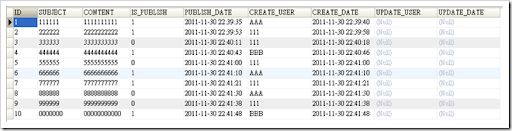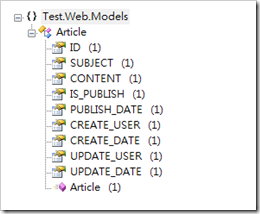`public class Article`
`{`
`    public string ID`
`    {`
`        get;`
`        set;`
`    }`
` `
`    public string SUBJECT`
`    {`
`        get;`
`        set;`
`    }`
` `
`    public string CONTENT`
`    {`
`        get;`
`        set;`
`    }`
` `
`    public bool IS_PUBLISH`
`    {`
`        get;`
`        set;`
`    }`
` `
`    public DateTime PUBLISH_DATE`
`    {`
`        get;`
`        set;`
`    }`
` `
`    public string CREATE_USER`
`    {`
`        get;`
`        set;`
`    }`
` `
`    public DateTime CREATE_DATE`
`    {`
`        get;`
`        set;`
`    }`
` `
`    public string UPDATE_USER`
`    {`
`        get;`
`        set;`
`    }`
` `
`    public DateTime? UPDATE_DATE`
`    {`
`        get;`
`        set;`
`    }`
` `
`    public Article()`
`    {`
`        this.IS_PUBLISH = false;`
`        this.CREATE_DATE = DateTime.Now;`
`    }`
`}`

`/// <summary>`
`/// Finds all.`
`/// </summary>`
`/// <returns></returns>`
`public List<Article> FindAll()`
`{`
`  string sql = " select * from ARTICLE order by CREATE_DATE asc ";`
` `
`  using (OracleConnection connection = new OracleConnection(this.connection_string))`
`  using (OracleCommand command = new OracleCommand(sql, connection))`
`  {`
`    try`
`    {`
`      if (connection.State != ConnectionState.Open)`
`      {`
`        connection.Open();`
`      }  `
` `
`      List<Article> data = new List<Article>();`
` `
`      using (OracleDataReader reader = command.ExecuteReader(CommandBehavior.CloseConnection))`
`      {`
`        while (reader.Read())`
`        {`
`          Article item = new Article();`
` `
`          item.ID = DBNull.Value.Equals(reader["ID"]) `
`            ? string.Empty `
`            : reader["ID"].ToString();`
`            `
`          item.SUBJECT = DBNull.Value.Equals(reader["SUBJECT"]) `
`            ? string.Empty `
`            : reader["SUBJECT"].ToString();`
`            `
`          item.CONTENT = DBNull.Value.Equals(reader["CONTENT"]) `
`            ? string.Empty `
`            : reader["CONTENT"].ToString();`
`            `
`          item.IS_PUBLISH = DBNull.Value.Equals(reader["IS_PUBLISH"]) `
`            ? false `
`            : reader["IS_PUBLISH"].ToString().Equals("1");`
`          `
`          item.PUBLISH_DATE = DBNull.Value.Equals(reader["PUBLISH_DATE"]) `
`            ? DateTime.MaxValue `
`            : Convert.ToDateTime(reader["PUBLISH_DATE"]);`
`          `
`          item.CREATE_USER = DBNull.Value.Equals(reader["CREATE_USER"]) `
`            ? string.Empty `
`            : reader["CREATE_USER"].ToString();`
`          `
`          item.CREATE_DATE = DBNull.Value.Equals(reader["CREATE_DATE"]) `
`            ? DateTime.Now `
`            : Convert.ToDateTime(reader["CREATE_DATE"]);`
`          `
`          item.UPDATE_USER = DBNull.Value.Equals(reader["UPDATE_USER"]) `
`            ? string.Empty `
`            : reader["UPDATE_USER"].ToString();`
` `
`          if (DBNull.Value.Equals(reader["UPDATE_DATE"]))`
`          {`
`            item.UPDATE_DATE = null;`
`          }`
`          else`
`          {`
`            item.UPDATE_DATE = Convert.ToDateTime(reader["UPDATE_DATE"]);  `
`          }`
`          data.Add(item);`
`        }`
`      }`
`      return data;`
`    }`
`    catch (Exception ex)`
`    {`
`      throw;`
`    }`
`  }`
`}`

`public ActionResult Index()`
`{`
`  DataAccess1 dataAccess1 = new DataAccess1();`
`  `
`  var data = dataAccess1.FindAll();`
`  ViewData["Count"] = data.Count;`
`  ViewData.Model = data;`
` `
`  return View();`
`}`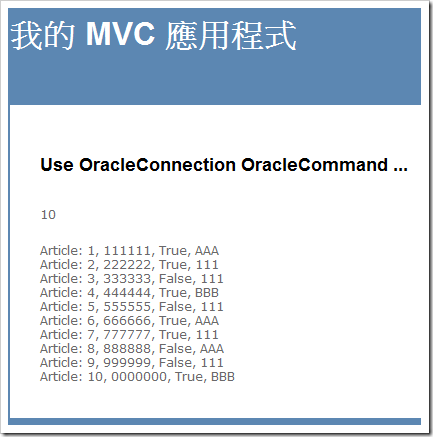`/// <summary>`
`/// Reads the object.`
`/// </summary>`
`/// <param name="reader">The reader.</param>`
`/// <returns></returns>`
`private Article ReadObject(IDataReader reader)`
`{`
`  Article item = new Article();`
` `
`  item.ID = DBNull.Value.Equals(reader["ID"]) `
`    ? string.Empty `
`    : reader["ID"].ToString();`
` `
`  item.SUBJECT = DBNull.Value.Equals(reader["SUBJECT"]) `
`    ? string.Empty : reader["SUBJECT"].ToString();`
` `
`  item.CONTENT = DBNull.Value.Equals(reader["CONTENT"]) `
`    ? string.Empty `
`    : reader["CONTENT"].ToString();`
` `
`  item.IS_PUBLISH = DBNull.Value.Equals(reader["IS_PUBLISH"]) `
`    ? false `
`    : reader["IS_PUBLISH"].ToString().Equals("1");`
` `
`  item.PUBLISH_DATE = DBNull.Value.Equals(reader["PUBLISH_DATE"]) `
`    ? DateTime.MaxValue `
`    : Convert.ToDateTime(reader["PUBLISH_DATE"]);`
` `
`  item.CREATE_USER = DBNull.Value.Equals(reader["CREATE_USER"]) `
`    ? string.Empty `
`    : reader["CREATE_USER"].ToString();`
` `
`  item.CREATE_DATE = DBNull.Value.Equals(reader["CREATE_DATE"]) `
`    ? DateTime.Now `
`    : Convert.ToDateTime(reader["CREATE_DATE"]);`
` `
`  item.UPDATE_USER = DBNull.Value.Equals(reader["UPDATE_USER"]) `
`    ? string.Empty `
`    : reader["UPDATE_USER"].ToString();`
` `
`  if (DBNull.Value.Equals(reader["UPDATE_DATE"]))`
`  {`
`    item.UPDATE_DATE = null;`
`  }`
`  else`
`  {`
`    item.UPDATE_DATE = Convert.ToDateTime(reader["UPDATE_DATE"]);`
`  }`
`  return item;`
`}`
` `

`/// <summary>`
`/// Finds the one.`
`/// </summary>`
`/// <param name="id">The id.</param>`
`/// <returns></returns>`
`public Article FindOne(string id)`
`{`
`  string sql = " select * from ARTICLE Where ID = :ID ";`
` `
`  using (OracleConnection connection = new OracleConnection(this.connection_string))`
`  using (OracleCommand command = new OracleCommand(sql, connection))`
`  {`
`    try`
`    {`
`      if (connection.State != ConnectionState.Open)`
`      {`
`        connection.Open();`
`      }`
` `
`      command.Parameters.Add("ID", id);`
` `
`      using (OracleDataReader reader = command.ExecuteReader(CommandBehavior.CloseConnection))`
`      {`
`        if (reader.Read())`
`        {`
`          return this.ReadObject(reader);`
`        }`
`      }`
`      return null;`
`    }`
`    catch (Exception ex)`
`    {`
`      throw;`
`    }`
`  }`
`}`
` `

`/// <summary>`
`/// Finds the by create user.`
`/// </summary>`
`/// <param name="user">The user.</param>`
`/// <returns></returns>`
`public List<Article> FindByCreateUser(string user)`
`{`
`  string sql = @" select * from ARTICLE where CREATE_USER = :CREATE_USER order by CREATE_DATE asc ";`
` `
`  using (OracleConnection connection = new OracleConnection(this.connection_string))`
`  using (OracleCommand command = new OracleCommand(sql, connection))`
`  {`
`    try`
`    {`
`      if (connection.State != ConnectionState.Open)`
`      {`
`        connection.Open();`
`      }`
` `
`      command.Parameters.Add("CREATE_USER", user);`
` `
`      using (OracleDataReader reader = command.ExecuteReader(CommandBehavior.CloseConnection))`
`      {`
`        List<Article> articles = new List<Article>();`
` `
`        while (reader.Read())`
`        {`
`          articles.Add(this.ReadObject(reader));`
`        }`
`        return articles;`
`      }`
`    }`
`    catch (Exception ex)`
`    {`
`      throw;`
`    }`
`  }`
`}`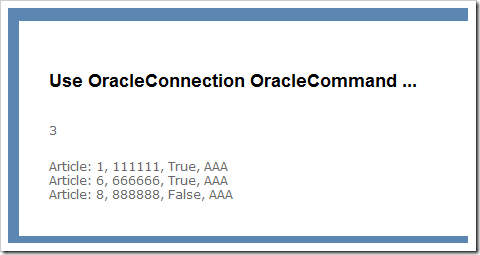FindByCondition() 方法，傳入的參數為 Dictionary<string, object>，Dictionary 的 Key 為表格的欄位名稱，Dictionaryt 的 Value 就是查詢值，

`/// <summary>`
`/// Finds the by conditions.`
`/// </summary>`
`/// <param name="columnValue">The column value.</param>`
`/// <returns></returns>`
`public List<Article> FindByConditions(Dictionary<string, object> columnValue)`
`{`
`  //Prepare Where Clause and OracleParameter`
` `
`  List<string> whereClause = new List<string>();`
`  List<OracleParameter> parameters = new List<OracleParameter>();`
` `
`  foreach (var item in columnValue)`
`  {`
`    if (!item.Key.Equals(string.Empty) && !item.Value.Equals(null))`
`    {`
`      whereClause.Add(string.Format(@"{0} = :{0}", item.Key));`
`      parameters.Add(new OracleParameter(item.Key, item.Value));`
`    }`
`  }`
`       `
`  string sql = string.Format(@" select * from Article where {0} order by CREATE_DATE desc ", string.Join(" and  ", whereClause.ToArray()));`
` `
`  using (OracleConnection connection = new OracleConnection(this.connection_string))`
`  using (OracleCommand command = new OracleCommand(sql, connection))`
`  {`
`    try`
`    {`
`      if (connection.State != ConnectionState.Open)`
`      {`
`        connection.Open();`
`      }`
` `
`      command.Parameters.AddRange(parameters.ToArray());`
` `
`      using (OracleDataReader reader = command.ExecuteReader(CommandBehavior.CloseConnection))`
`      {`
`        List<Article> articles = new List<Article>();`
` `
`        while (reader.Read())`
`        {`
`          articles.Add(this.ReadObject(reader));`
`        }`
`        return articles;`
`      }`
`    }`
`    catch (Exception ex)`
`    {`
`      throw;`
`    }`
`  }  `
` `
`}`
` `

`public ActionResult About()`
`{`
`  DataAccess1 dataAccess = new DataAccess1();`
` `
`  Dictionary<string, object> conditions = new Dictionary<string, object>();`
`  conditions.Add("IS_PUBLISH", 1);`
`  conditions.Add("CREATE_USER", "AAA");`
` `
`  var data = dataAccess.FindByConditions(conditions);`
` `
`  ViewData["Count"] = data.Count;`
`  ViewData.Model = data;`
` `
`  return View();`
`}`
` `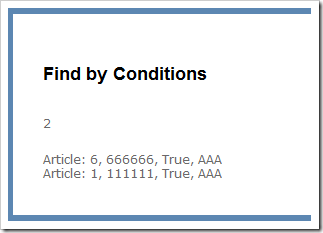### Microsoft Enterprise Library 5.0

http://msdn.microsoft.com/en-us/library/ff632023.aspx

http://entlib.codeplex.com/

Enterprise Library 5.0 Class Library on MSDN

Microsoft Enterprise Library 5.0 Hands-on Labs

Microsoft Enterprise Library 5.0 Extensibility Hands-on Labs

### The Data Access Application Block

http://msdn.microsoft.com/en-us/library/ff664408(v=PandP.50).aspx

#### 使用 Nuget 加入 Enterprise Library 的 Data Access Application Block 參考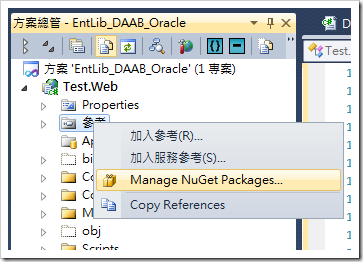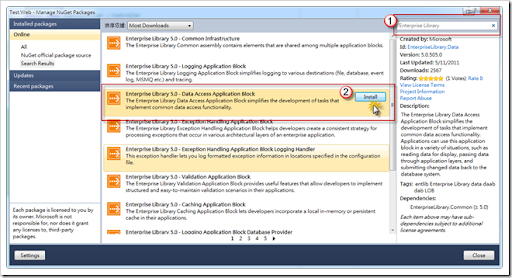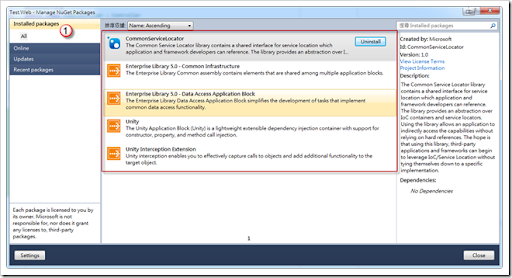#### 使用Enterprise Library的Data Access Application Block方式來讀取Oracle資料庫中的資料

`using Microsoft.Practices.EnterpriseLibrary.Common.Configuration;`
`using Microsoft.Practices.EnterpriseLibrary.Data;`

`private Database _db;`
`protected Database db`
`{`
`    get`
`    {`
`        if (_db == null)`
`        {`
`            _db = EnterpriseLibraryContainer.Current.GetInstance<Database>("OracleNorthwind");`
`        }`
`        return _db;`
`    }`
`}`

FindAll() 方法：

`/// <summary>`
`/// Finds all.`
`/// </summary>`
`/// <returns></returns>`
`public List<Article> FindAll()`
`{`
`  string sql = " select * from ARTICLE order by CREATE_DATE asc ";`
` `
`  using (DbCommand command = db.GetSqlStringCommand(sql))`
`  {`
`    try`
`    {`
`      List<Article> data = new List<Article>();`
` `
`      using (IDataReader reader = db.ExecuteReader(command))`
`      {`
`        while (reader.Read())`
`        {`
`          data.Add(this.ReadObject(reader));`
`        }`
`      }`
`      return data;`
`    }`
`    catch (Exception ex)`
`    {`
`      throw;`
`    }`
`  }`
`}`

FindAll() 方法使用：

`public ActionResult Index()`
`{`
`  DataAccess2 dataAccess = new DataAccess2();`
` `
`  var data = dataAccess.FindByCreateUser("AAA");`
`  ViewData["Count"] = data.Count;`
`  ViewData.Model = data;`
` `
`  return View();`
`}`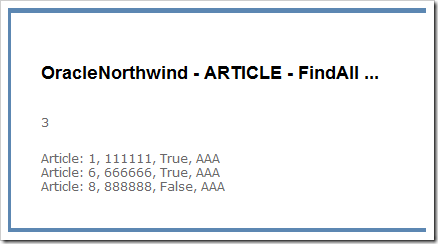`/// <summary>`
`/// Finds the by create user.`
`/// </summary>`
`/// <param name="user">The user.</param>`
`/// <returns></returns>`
`public List<Article> FindByCreateUser(string user)`
`{`
`  string sql = @" select * from ARTICLE where CREATE_USER = :CREATE_USER order by CREATE_DATE asc ";`
` `
`  using (DbCommand command = db.GetSqlStringCommand(sql))`
`  {`
`    command.Parameters.Add(new OracleParameter("CREATE_USER", user));`
` `
`    try`
`    {`
`      using (IDataReader reader = db.ExecuteReader(command))`
`      {`
`        List<Article> articles = new List<Article>();`
` `
`        while (reader.Read())`
`        {`
`          articles.Add(this.ReadObject(reader));`
`        }`
`        return articles;`
`      }`
`    }`
`    catch (Exception ex)`
`    {`
`      throw;`
`    }`
`  }`
`}`

#### 相關的詳細資料與操作可以參考MSDN的說明：

Returning Data as Objects for Client Side Querying：http://msdn.microsoft.com/en-us/library/ff664431(v=PandP.50).aspx

IRowMapper的實作就只有一個方法，MapRow，傳入的參數型別為IDataRecord，

`public class SomeObjectMapper : IRowMapper<Article>`
`{`
`    public Article MapRow(IDataRecord row)`
`    {`
`        throw new NotImplementedException();`
`    }`
`}`

IDataRecord Interface：http://msdn.microsoft.com/en-us/library/system.data.idatarecord.aspx

ArticleMapper類別

`//=========================================================================================`
`//IRowMapper`
` `
`public class ArticleMapper : IRowMapper<Article>`
`{`
`  public Article MapRow(IDataRecord reader)`
`  {`
`    Article item = new Article();`
` `
` `
`    item.ID = DBNull.Value.Equals(reader["ID"]) `
`        ? string.Empty `
`        : reader["ID"].ToString();`
` `
`    item.SUBJECT = DBNull.Value.Equals(reader["SUBJECT"]) `
`        ? string.Empty `
`        : reader["SUBJECT"].ToString();`
` `
`    item.CONTENT = DBNull.Value.Equals(reader["CONTENT"]) `
`        ? string.Empty `
`        : reader["CONTENT"].ToString();`
` `
`    item.IS_PUBLISH = DBNull.Value.Equals(reader["IS_PUBLISH"]) `
`        ? false `
`        : reader["IS_PUBLISH"].ToString().Equals("1");`
` `
`    item.PUBLISH_DATE = DBNull.Value.Equals(reader["PUBLISH_DATE"]) `
`        ? DateTime.MaxValue `
`        : Convert.ToDateTime(reader["PUBLISH_DATE"]);`
` `
`    item.CREATE_USER = DBNull.Value.Equals(reader["CREATE_USER"]) `
`        ? string.Empty `
`        : reader["CREATE_USER"].ToString();`
` `
`    item.CREATE_DATE = DBNull.Value.Equals(reader["CREATE_DATE"]) `
`        ? DateTime.Now `
`        : Convert.ToDateTime(reader["CREATE_DATE"]);`
` `
`    item.UPDATE_USER = DBNull.Value.Equals(reader["UPDATE_USER"]) `
`        ? string.Empty `
`        : reader["UPDATE_USER"].ToString();`
` `
`    if (DBNull.Value.Equals(reader["UPDATE_DATE"]))`
`    {`
`      item.UPDATE_DATE = null;`
`    }`
`    else`
`    {`
`      item.UPDATE_DATE = Convert.ToDateTime(reader["UPDATE_DATE"]);`
`    }`
` `
`    return item;`
`  }`
`}`

`/// <summary>`
`/// Finds all.`
`/// </summary>`
`/// <returns></returns>`
`public List<Article> FindAll()`
`{`
`  string sql = " select * from ARTICLE order by CREATE_DATE asc ";`
` `
`  try`
`  {`
`    DataAccessor<Article> accessor = db.CreateSqlStringAccessor<Article>(sql, new ArticleMapper());`
`    var data = accessor.Execute().ToList();`
`    return data;`
`  }`
`  catch (Exception ex)`
`  {`
`    throw;`
`  }`
`}`

`public class SomeDataParameterMapper : IParameterMapper`
`{`
`    public void AssignParameters(DbCommand command, object[] parameterValues)`
`    {`
`        throw new NotImplementedException();`
`    }`
`}`

`string sql = @" select * from ARTICLE where CREATE_USER = :CREATE_USER order by CREATE_DATE asc ";`

`public class FindByCreateUserParameterMapper : IParameterMapper`
`{`
`    public void AssignParameters(DbCommand command, object[] parameterValues)`
`    {`
`        command.Parameters.Add(new OracleParameter("CREATE_USER", parameterValues));`
`    }`
`}`

FindByCreateUser()方法：

`/// <summary>`
`/// Finds the by create user.`
`/// </summary>`
`/// <param name="user">The user.</param>`
`/// <returns></returns>`
`public List<Article> FindByCreateUser(string user)`
`{`
`    string sql = @" select * from ARTICLE where CREATE_USER = :CREATE_USER order by CREATE_DATE asc ";`
` `
`    try`
`    {`
`        DataAccessor<Article> accessor = db.CreateSqlStringAccessor<Article>(sql, new FindByCreateUserParameterMapper(), new ArticleMapper());`
`        var data = accessor.Execute(new object[] { user }).ToList();`
`        return data;`
`    }`
`    catch (Exception ex)`
`    {`
`        throw;`
`    }`
`}`
` `

CreateSqlStringAccessor() 方法還有以下的一個多載：

CreateSqlStringAccessor(TResult) Method (Database, String, IParameterMapper, IRowMapper(TResult))

（其實比較好的方式就是看需求來決定要使用哪一種，要動態增減參數的時候，還是使用DAAB原本的方法）

#### 5 則留言:

1.請教版主,
使用 EntLib 時，當遇到sqlStatement 中有 where in ('A','B',...) 之類的語法時，在設計 ParameterMapper 總是不順，
對於這類有否其他更好的撰寫方式?

1.這個我這邊沒有什麼比較好的建議，我還是使用一個一個的對映做處理，不然就是跑個迴圈，然後組成 in clause 裡所使用的字串然後帶入 parameter 裡，可以參靠下面文章的作法（部知道這是不是符合你的情形，不用看最下面 LINQ 方式，看 ADO.NET 的方式）
Parameterized IN clauses with ADO.NET and LINQ

2.請問，有時候會碰到連線字串是需要加密的情況，這部分 EntLib 要怎麼處理?

1.Hello, 你好
如果是使用 EntLib5 以前的版本，可以使用 configuration tools 然後搭配 Cryptography Application Blocks 來完成，可參考以下連結（http://msdn.microsoft.com/en-us/library/ff664594(v=pandp.50).aspx），或是查詢「Enterprise Library Cryptography Application Blocks」
http://www.cnblogs.com/huangcong/archive/2010/05/28/1746634.html
http://www.cnblogs.com/huangcong/archive/2010/05/29/1747015.html

如果是使用 EntLib 6 的話，因為已將 Cryptography Application Blocks 移出去了，不過官方文件還是有提供如何對設定檔加密處理的文章，可以看下的連結：
MSDN - Enterprise Library 6 - Appendix B - Encrypting Configuration Files
http://msdn.microsoft.com/en-us/library/dn440730(v=pandp.60).aspx

3.謝謝版主